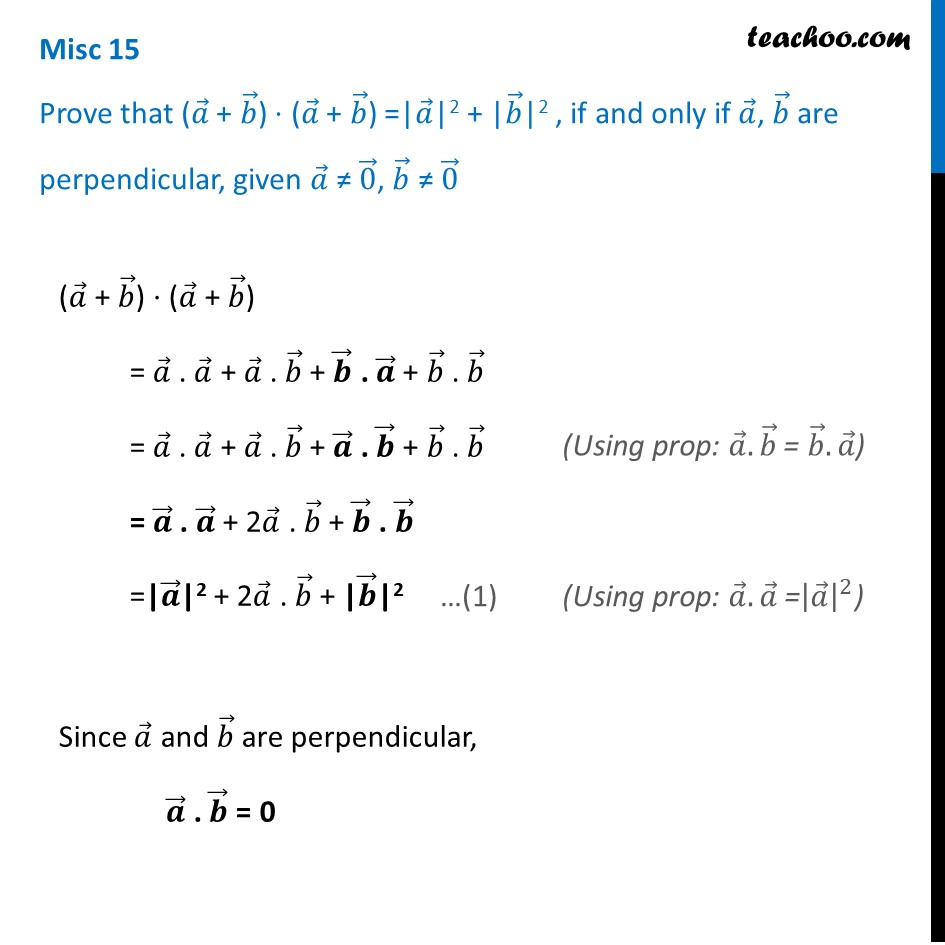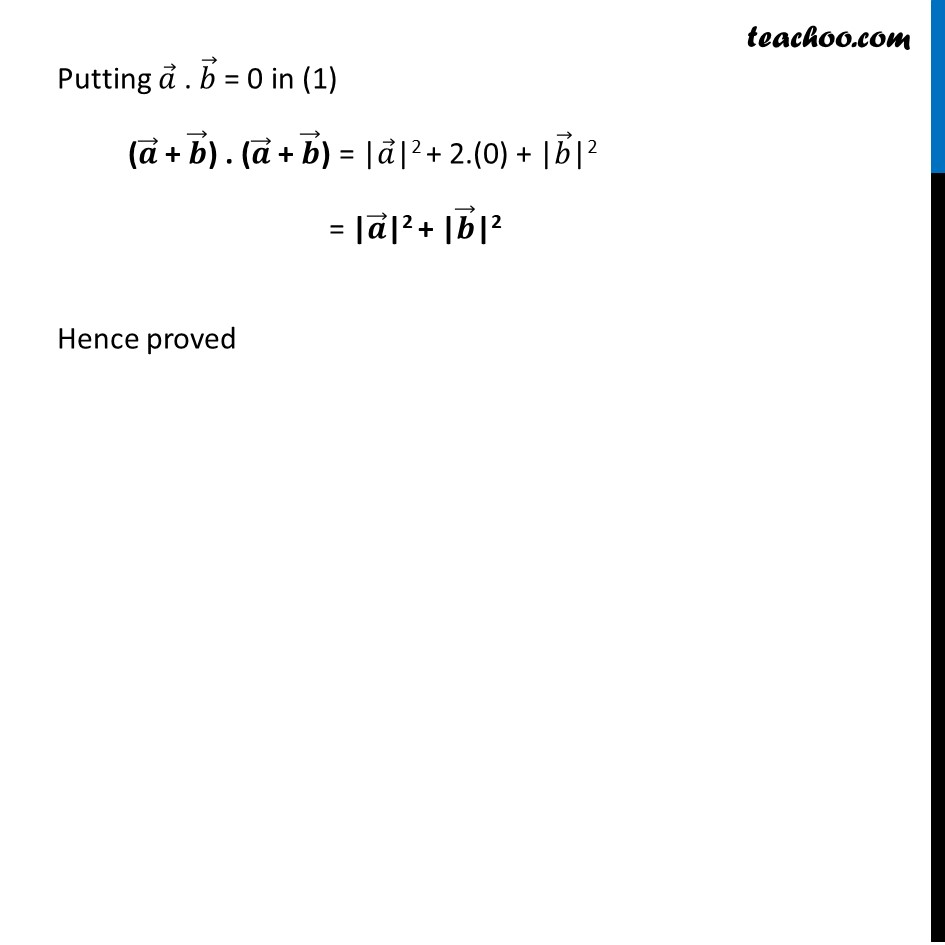Scalar product - Solving

Chapter 10 Class 12 Vector Algebra
Concept wiseLearn in your speed, with individual attention - Teachoo Maths 1-on-1 Class

### Transcript

Misc 15 Prove that (𝑎 ⃗ + 𝑏 ⃗) ⋅ (𝑎 ⃗ + 𝑏 ⃗) =|𝑎 ⃗|2 + |𝑏 ⃗|2 , if and only if 𝑎 ⃗, 𝑏 ⃗ are perpendicular, given 𝑎 ⃗ ≠ 0 ⃗, 𝑏 ⃗ ≠ 0 ⃗ (𝑎 ⃗ + 𝑏 ⃗) ⋅ (𝑎 ⃗ + 𝑏 ⃗) = 𝑎 ⃗ . 𝑎 ⃗ + 𝑎 ⃗ . 𝑏 ⃗ + 𝒃 ⃗ . 𝒂 ⃗ + 𝑏 ⃗ . 𝑏 ⃗ = 𝑎 ⃗ . 𝑎 ⃗ + 𝑎 ⃗ . 𝑏 ⃗ + 𝒂 ⃗ . 𝒃 ⃗ + 𝑏 ⃗ . 𝑏 ⃗ = 𝒂 ⃗ . 𝒂 ⃗ + 2𝑎 ⃗ . 𝑏 ⃗ + 𝒃 ⃗ . 𝒃 ⃗ =|𝒂 ⃗|2 + 2𝑎 ⃗ . 𝑏 ⃗ + |𝒃 ⃗|2 Since 𝑎 ⃗ and 𝑏 ⃗ are perpendicular, 𝒂 ⃗ . 𝒃 ⃗ = 0 (Using prop: 𝑎 ⃗.𝑏 ⃗ = 𝑏 ⃗.𝑎 ⃗) (Using prop: 𝑎 ⃗.𝑎 ⃗ =|𝑎 ⃗ |^2) Putting 𝑎 ⃗ . 𝑏 ⃗ = 0 in (1) (𝒂 ⃗ + 𝒃 ⃗) . (𝒂 ⃗ + 𝒃 ⃗) = |𝑎 ⃗|2 + 2.(0) + |𝑏 ⃗|2 = |𝒂 ⃗|2 + |𝒃 ⃗|2 Hence proved